• PyTorch基础入门三：PyTorch搭建多项式回归模型  1）理论简介 对于一般的线性回归模型，由于该函数拟合出来的是一条直线，所以精度欠佳，我们可以考虑多项式回归来拟合更多的模型。所谓多项式回归，其本质也是线性...
• 本案例使用房价预测的数据，使用Python做了线性回归、二次回归和三次回归，并分别得出三者的模型拟合得分，来探究针对本案例的模型拟合效果。也侧面分析了欠拟合、过拟合的现象。
• 主要介绍了详解Pytorch 使用Pytorch拟合多项式(多项式回归),小编觉得挺不错的，现在分享给大家，也给大家做个参考。一起跟随小编过来看看吧
• 3. 多项式模型 (一元多次方程) 3.1 多项式拟合 在有些数据分布中，使用一条曲线比直线能更好拟合数据，这就需要用到多项式拟合。如下图所示分布： 多项式的一般形式： y=p0xn+p1xn−1+p2xn−2+p3xn−3+...+pn y=p_{...
3. 多项式模型 (一元多次方程)
3.1 多项式拟合
在有些数据分布中，使用一条曲线比直线能更好拟合数据，这就需要用到多项式拟合。如下图所示分布：多项式的一般形式：

y

=

p

0

x

n

+

p

1

x

n

−

1

+

p

2

x

n

−

2

+

p

3

x

n

−

3

+

.

.

.

+

p

n

y=p_{0}x^n + p_{1}x^{n-1} + p_{2}x^{n-2} + p_{3}x^{n-3} +...+p_{n}

多项式拟合的目的是为了找到一组

p

0

,

p

1

,

.

.

.

,

p

n

p_0, p_1, ..., p_n

，使得拟合方程尽可能的与实际样本数据相符合。
假设拟合得到的多项式如下：

f

(

x

)

=

p

0

x

n

+

p

1

x

n

−

1

+

p

2

x

n

−

2

+

p

3

x

n

−

3

+

.

.

.

+

p

n

f(x)=p_{0}x^n + p_{1}x^{n-1} + p_{2}x^{n-2} + p_{3}x^{n-3} +...+p_{n}

则拟合函数与真实结果的差方如下：

l

o

s

s

=

(

y

1

−

f

(

x

1

)

)

2

+

(

y

2

−

f

(

x

2

)

)

2

+

.

.

.

+

(

y

n

−

f

(

x

n

)

)

2

loss = (y_1-f(x_1))^2 + (y_2-f(x_2))^2 + ... + (y_n-f(x_n))^2

那么多项式拟合的过程即为求取一组

p

0

,

p

1

,

.

.

.

,

p

n

p_0, p_1, ..., p_n

, 使得loss的值最小。在程序中，多项式可以表示为一个数组，格式如下：
f = [-6, 3, 8, 1]

表示多项式为：

y

=

−

6

x

3

+

3

x

2

+

8

x

+

1

y=-6x^3 + 3x^2 + 8x + 1

3.2 多项式API

Python中, 可以用 numpy.polyfit() 函数进行多项式拟合

X = [x1, x2, ..., xn]
Y = [y1, y2, ..., yn]
# 根据一组样本，并给出最高次幂，求出拟合系数
np.polyfit(X, Y, 最高次幂)	# 得到的是一个一维数组


其他多项式运算相关函数

# 根据拟合系数与自变量求出拟合值, 由此可得拟合曲线坐标样本数据 [X, Y']
np.polyval(P, X)->Y'

# 多项式函数求导，根据拟合系数求出多项式函数导函数的系数
np.polyder(P)->Q

# 已知多项式系数Q 求多项式函数的根（与x轴交点的横坐标, 即波峰波谷）
xs = np.roots(Q)

# 两个多项式函数的差函数（对应系数相减）的系数（可以通过差函数的根求取两个曲线的交点）
Q = np.polysub(P1, P2)

3.3 苹果股价案例

打开文件, 查看苹果股票的日期与收盘价格

import pandas as pd
import numpy as np

names=['dates','close'])		# 日期 & 当日收市股价
print(data)
"""
dates		close
0	2011-01-28	336.10
1	2011-01-31	339.32
2	2011-02-01	345.03
3	2011-02-02	344.32
4	2011-02-03	343.44
...		...		...
27	2011-03-09	352.47
28	2011-03-10	346.67
29	2011-03-11	351.99
"""


为了方便进行数学运算, 需要把日期改为数字格式, 可以用2011-01-01为0, 计算每个日期距离2011-01-01的天数为日期值 delta

data['delta'] = data['dates'] - pd.to_datetime('2011-01-01')
data['delta'] = data['delta'].dt.days
print(data)
"""
dates		close	delta
0	2011-01-28	336.10	27
1	2011-01-31	339.32	30
2	2011-02-01	345.03	31
3	2011-02-02	344.32	32
...		...		...		...
"""


画出close和delta的图表

data.plot(x='delta', y='close')a) 多项式拟合

针对股票价格, 用polyfit()函数进行多项式拟合

# polyfit函数, 需要(x数据, y数据, deg=项数)三个必要参数,
p = np.polyfit(data['delta'], data['close'], 5)
print(p)
# array([-1.97919911e-05,  4.66447608e-03, -4.27249275e-01,  1.89446747e+01, -4.05027302e+02,  3.67749979e+03])
# 得到的是多项式函数的系数

b) 计算拟合值

根据多项式函数, 可以用polyval()函数计算拟合值
我们可以计算200个拟合值, 画出曲线图 (折线图的点变多了, 就变成曲线图了~)

# 将delta从最小值到最大值之间平均分割200个数, 得到一个一维数组
xs = np.linspace(data['delta'].min(), data['delta'].max(), 200)
# linspace函数是创建数值序列的工具.
# 需要指定间隔起始点、终止端，以及指定分隔值总数（包括起始点和终止点）；最终函数返回间隔类均匀分布的数值序列

# polyval函数, 根据拟合系数与自变量求出拟合值, 由此可得拟合曲线坐标样本数据
# 根据函数系数p(用polyfit函数计算得到), 以及x的值序列xs(用linspace函数计算得到), 求出y值序列ys
ys = np.polyval(p, xs)

# 画出源数据图
plt.plot(data['delta'], data['close'])
# 画出多项式拟合图
plt.plot(xs, ys)c) 欠拟合 & 过拟合
欠拟合:
欠拟合是指模型拟合程度不高，数据距离拟合曲线较远，或指模型没有很好地捕捉到数据特征，不能够很好地拟合数据上图的拟合就较为欠缺
过拟合:
为了得到一致假设而使假设变得过度严格称为过拟合一个假设在训练数据上能够获得比其他假设更好的拟合， 但是在训练数据外的数据集上却不能很好地拟合数据，此时认为这个假设出现了过拟合的现象。出现这种现象的主要原因是训练数据中存在噪音或者训练数据太少

调整多项式的最高次幂来调整拟合度

p = np.polyfit(data['delta'], data['close'], 20)				# 将最高次幂调整为20
xs = np.linspace(data['delta'].min(), data['delta'].max(), 200)
ys = np.polyval(p, xs)
plt.plot(data['delta'], data['close'])
plt.plot(xs, ys)


根据下图可以看得出来, 多项式函数可以很好的拟合股价波动了, 但是在一些位置出现了过拟合继续调整多项式的最高次幂来找到最佳的拟合函数

p = np.polyfit(data['delta'], data['close'], 9)				# 将最高次幂调整为9
xs = np.linspace(data['delta'].min(), data['delta'].max(), 200)
ys = np.polyval(p, xs)
plt.plot(data['delta'], data['close'])
plt.plot(xs, ys)d) 函数趋势

得到某一时刻股价的趋势 (涨/跌) polyder函数可以根据拟合系数求出多项式函数导函数的系数

# polyder()函数用于多项式求导
q = np.polyder(p)

# 当x=46时, 判断是涨还是跌
# 将46放入导数函数
k = np.polyval(q, 46)
print(k)		# -1.9249425322705065
# k为负数表示, 当x=46时, 股价的趋势是下降的e) 波峰波谷

如何求出波峰波谷? ==> 函数切线斜率为0的位置 ==> 即导数函数的根

xs = np.roots(q)
print(xs)
# array([64.81228477, 54.26409333, 41.25554414, 28.20802013])
# 求出的是x轴的值线性函数或多项式函数只是对于已经发生的数据继续拟合, 无法用于预测未发生的

展开全文python 机器学习 数据分析 numpy 线性代数
• 多项式回归拟合、正则化1 多项式回归1.1 介绍1.2 回归实现2 拟合&正则化2.1拟合问题2.1.1 拟合出现的类型2.2 解决拟合出现的问题3 正则化3.1 介绍3.2 分类 1 多项式回归 1.1 介绍 在线性回归中，我们介绍了的...


多项式回归和拟合、正则化
1 多项式回归1.1 介绍1.2 回归实现
2 拟合&正则化2.1拟合问题2.1.1 拟合出现的类型2.2 解决拟合出现的问题

3 正则化3.1 介绍3.2 分类

1 多项式回归
1.1 介绍
在线性回归中，我们介绍了的是这种模型y = kX +b来拟合图形，但是也存在一些数据分析用线性拟合效果很差，或者说不适合用线性回归来拟合，如下图相对而言，用多项式就比较合适。多项式就是如同 y = a + bx^2 + c这种， y = a + bx^2 +cx^3 + d 等等
也就是多项式的模型一般是这样子的：1.2 回归实现
下面以这个数据做一个示例import numpy as np
import matplotlib.pyplot as plt
from sklearn.linear_model import LinearRegression
from sklearn.preprocessing import PolynomialFeatures
from numpy import genfromtxt

# 加载数据
data = genfromtxt("job.csv", delimiter=",")

x_data = data[1:, 1]
y_data = data[1:, 2]
plt.scatter(x_data, y_data)
plt.show()
# print(x_data, y_data)线性回归
# 一维变二维
x_data = x_data[:, np.newaxis]
y_data = y_data[:, np.newaxis]

# 创建并拟合模型
model = LinearRegression()
model.fit(x_data, y_data)

plt.scatter(x_data, y_data)
plt.plot(x_data, model.predict(x_data), 'r')
plt.show()多项式回归
# 定义多项式回归, degree的值可以调节多项式的特征
poly_reg = PolynomialFeatures(degree=3)
# 特征处理
x_poly = poly_reg.fit_transform(x_data)

# 定义回归模型
reg = LinearRegression()
reg.fit(x_poly, y_data)

plt.plot(x_data, y_data, 'b.')
plt.plot(x_data, reg.predict(x_poly), 'r')
plt.show()2 拟合&正则化
之前我们在模型那一块有讲过模型拟合会出现欠拟合、过拟合，今天我们来具体讲一下吧
2.1拟合问题
2.1.1 拟合出现的类型
过拟合：一个假设在训练数据上能够获得比其他假设更好的拟合， 但是在测试数据集上却不能很好地拟合数据，此时认为这个假设出现了过拟合的现象。(模型过于复杂)欠拟合：一个假设在训练数据上不能获得更好的拟合，并且在测试数据集上也不能很好地拟合数据，此时认为这个假设出现了欠拟合的现象。(模型过于简单)
回归中出现的拟合问题：分类拟合中出现的问题如下：2.2 解决拟合出现的问题
（1）欠拟合原因以及解决办法 原因：学习到数据的特征过少 解决办法： • 1）添加其他特征项，有时候我们模型出现欠拟合的时候是因为特征项不够导致的，可以添加其他特征项来很好地解决。例如，“组合”、“泛化”、“相关性”三类特征是特征添加的重要手段，无论在什么场景，都可以照葫芦画瓢，总会得到意想不到的效果。除上面的特征之外，“上下文特征”、“平台特征”等等，都可以作为特征添加的首选项。 • 2）添加多项式特征，这个在机器学习算法里面用的很普遍，例如将线性模型通过添加二次项或者三次项使模型泛化能力更强。
（2）过拟合原因以及解决办法 原因：原始特征过多，存在一些嘈杂特征， 模型过于复杂是因为模型尝试去兼顾各个测试数据点 解决办法： • 1）重新清洗数据，导致过拟合的一个原因也有可能是数据不纯导致的，如果出现了过拟合就需要我们重新清洗数据。 • 2）增大数据的训练量，还有一个原因就是我们用于训练的数据量太小导致的，训练数据占总数据的比例过小。 • 3）正则化 • 4）减少特征维度，防止维灾难
3 正则化
3.1 介绍
在学习的时候，数据提供的特征有些影响模型复杂度或者这个特征的数据点异常较多，所以算法在学习的时候尽量减少这个特征的影响（甚至删除某个特征的影响），这就是正则化。如图示例3.2 分类
L2正则化 1）作用：可以使得其中一些W（权重）的都很小，都接近于0，削弱某个特征的影响 2）优点：越小的参数说明模型越简单，越简单的模型则越不容易产生过拟合现象 3）Ridge回归 4）代价函数L1正则化 1）作用：可以使得其中一些W的值直接为0，删除这个特征的影响 2）LASSO回归 3）代价函数展开全文机器学习 python
• 很过数据是没有线性关系的==》非线性 =》多项式模拟非线性曲线 添加的特征是原来特征的线性组合==》解决非线性问题=》数据集升维 (PCA降维) 有时升维有时降维 ...多项式回归拟合非线性： 一个二次曲线...

很过数据是没有线性关系的==》非线性 =》多项式模拟非线性曲线
添加的特征是原来特征的线性组合==》解决非线性问题=》数据集升维      (PCA降维)  有时升维有时降维
=》x^2  x  虽然是同一特征 但我们把它x^2当做是另一个特征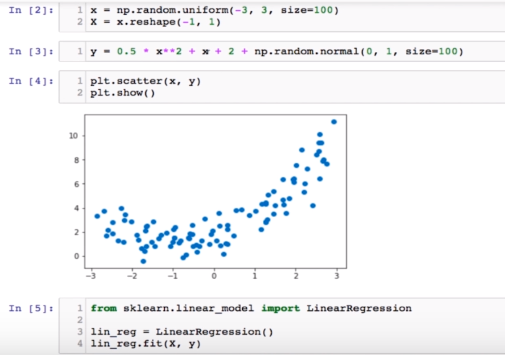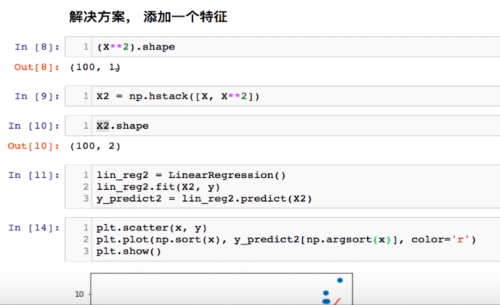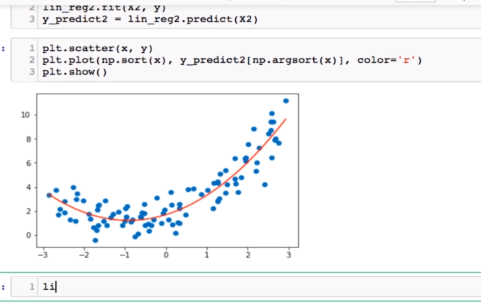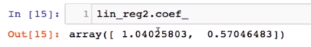Pipeline   创建多项式回归：  通过增加特征 依然使用使用线性回归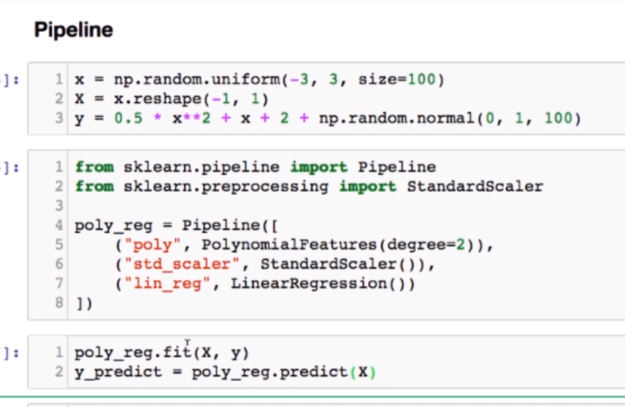多项式回归拟合非线性：
一个二次曲线   ==》过拟合  100次太复杂了  训练集上好  在验证集  和  测试集不好  欠拟合 1次不够
学习狗的图片：
有眼睛的是狗：只学习一部分特征 欠拟合
四爪 眼睛 ... 斑点 :所有符合的基础上又加上了 斑点   斑点只是一部分狗才有  可过拟合学出了斑点特征      太细节的特征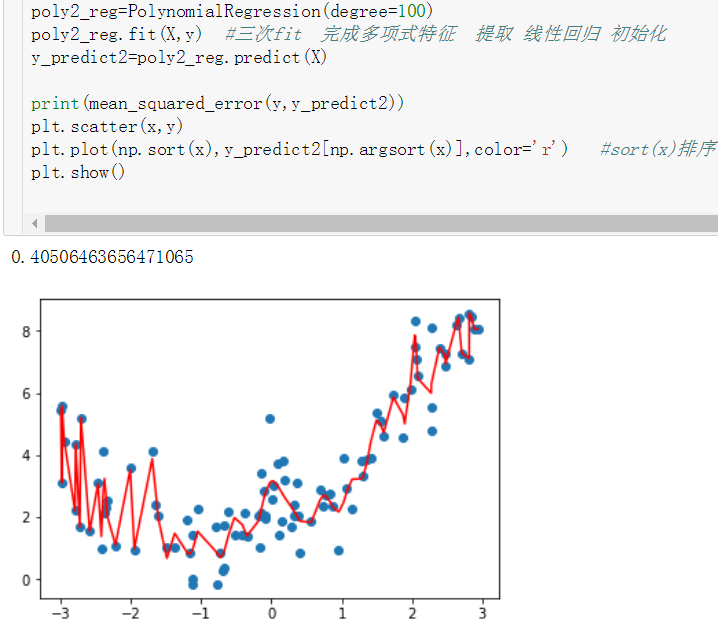泛化能力：由此及彼能力       根据训练得到的曲线         面对新的数据的能力
最终的模型最终能力不是拟合训练集 而是更好的拟合  测试集 新数据 =》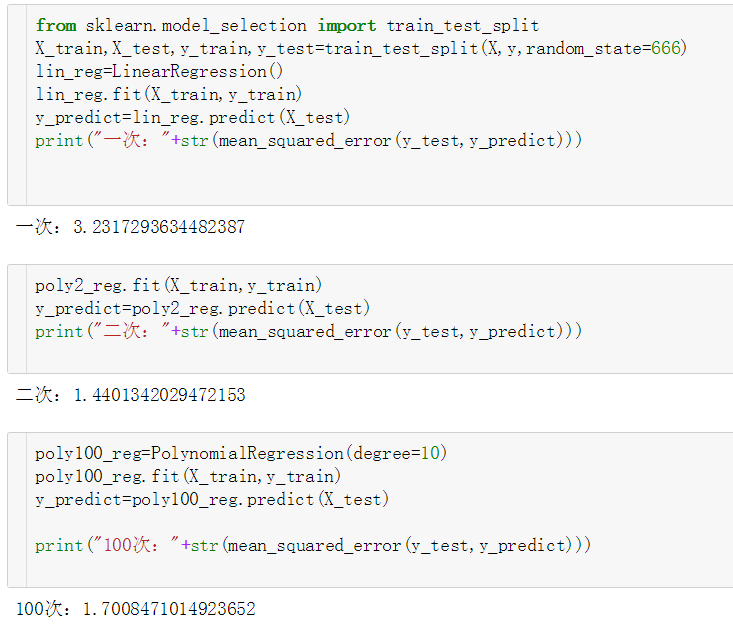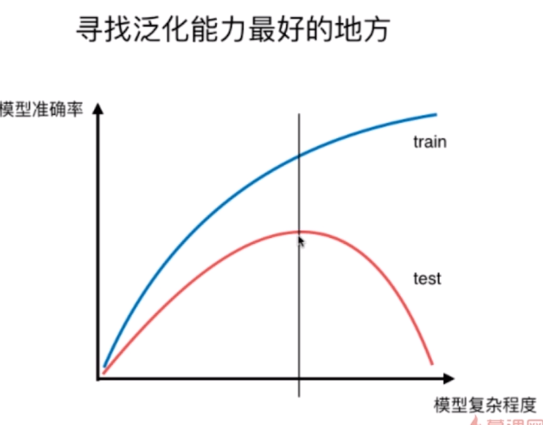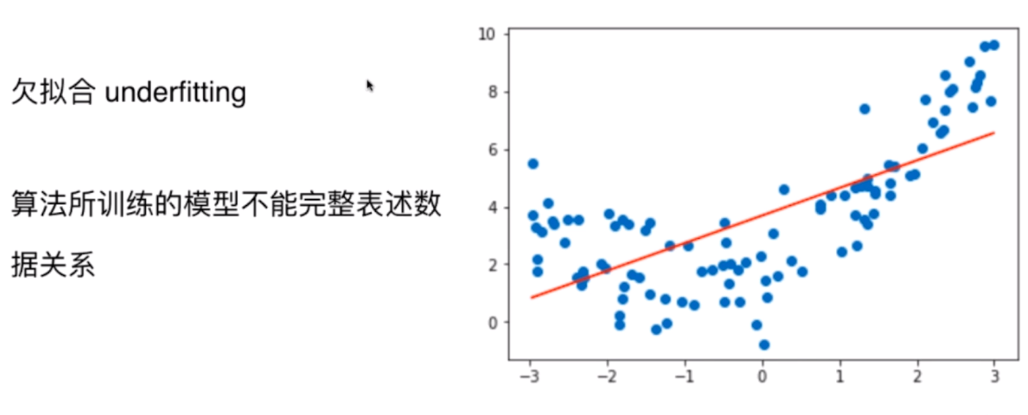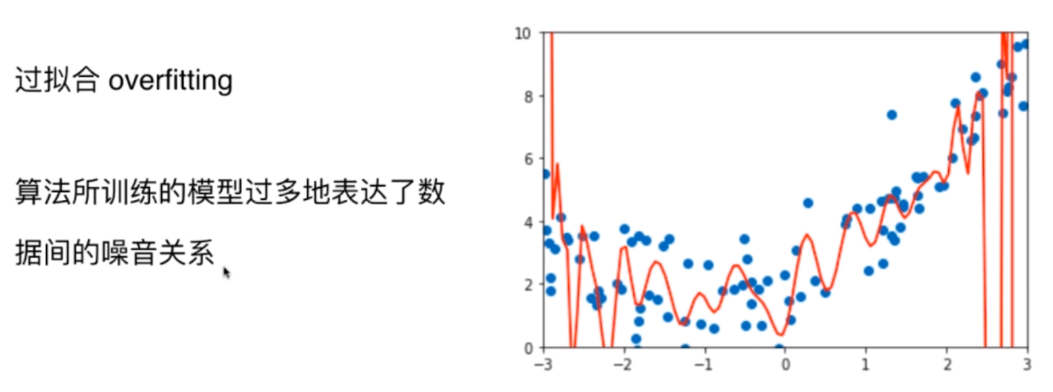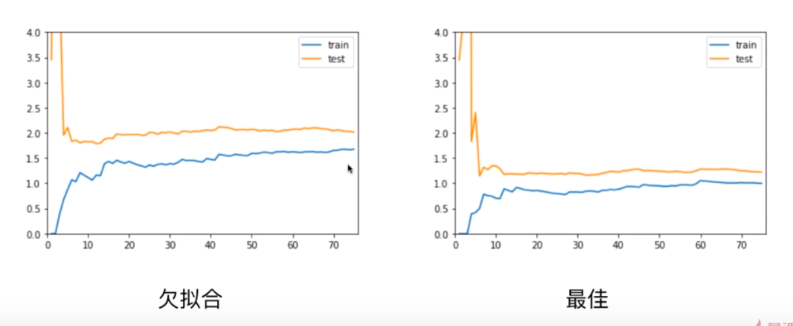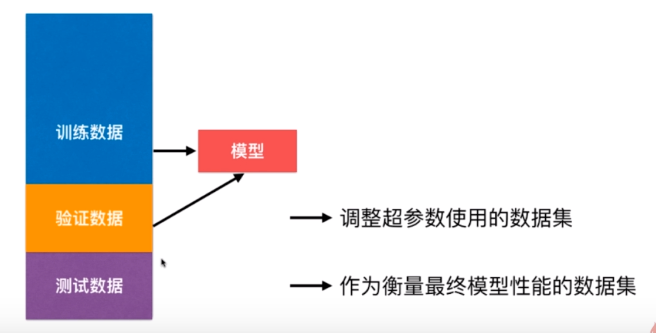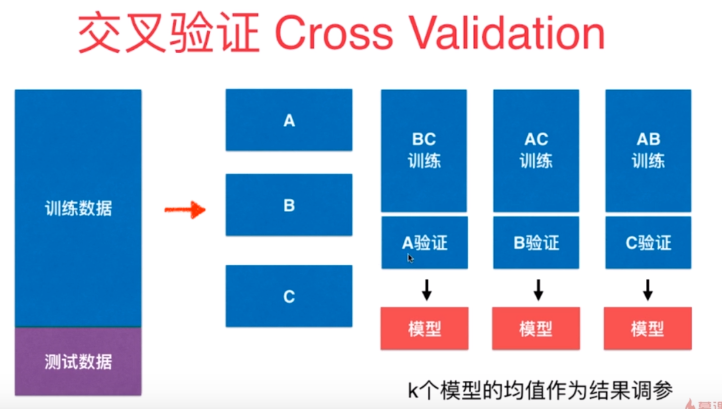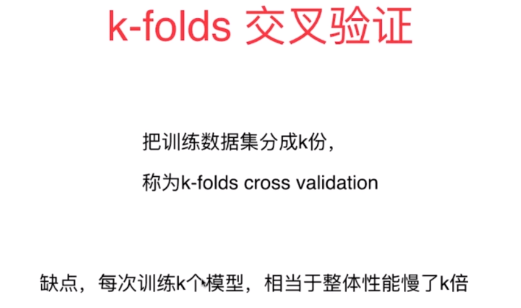train valitation test
欠拟合：训练 测试上dou不好     过拟合 ：训练上低  测试上高
为了防止   验证集中有极端数据   防止关于验证集的过拟合  ==》交叉验证
评价模型准确度更加靠谱：
==》每次都要训练k个模型  才确定一组参数  比正常多耗时K倍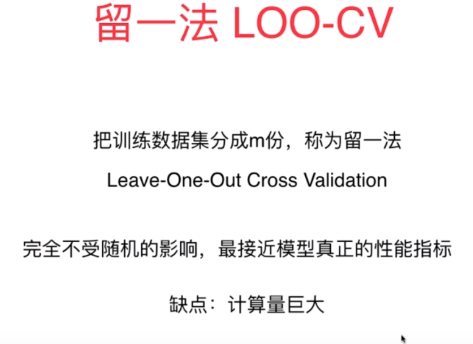M个样本分为  m分  用其中一份作为验证集 其他用于训练
高偏差：模型太偏离原问题  ==》    一些数据可能原本就不是线性的    如果非要用线性拟合的话就容易高偏差  》特征取得不好
我们假设数据是符合某个模型的一旦不符合极易出现高偏差  degree越小越简单 degree越大形状越奇怪引入的方差越大

高方差：泛化能力差    KNN 多依赖于数据==》非参数学习      k越小模型越复杂 方差可能越大

偏差 和 方差是可以避免的         不可避免的误差如 数据中有噪音
偏差：
1.用名字预测成绩  选取特征  离目标是高度不相关的
2.欠拟合
方差：过拟合  学习的过度  分的太细
1.降低模型复杂度
2.过拟合可能学到的太多的噪音数据
3.增加样本量   深度学习的条件是数据规模要足够的大  不然不如简单地模型
4.使用验证集   防止模型针对测试数据集过拟合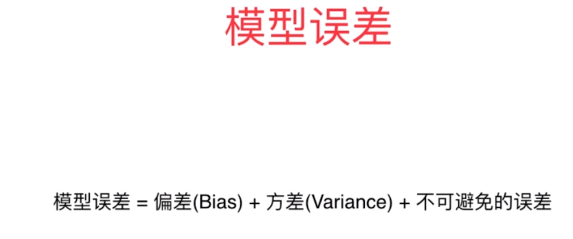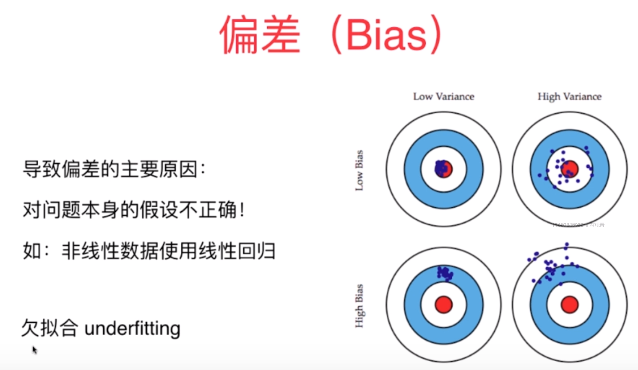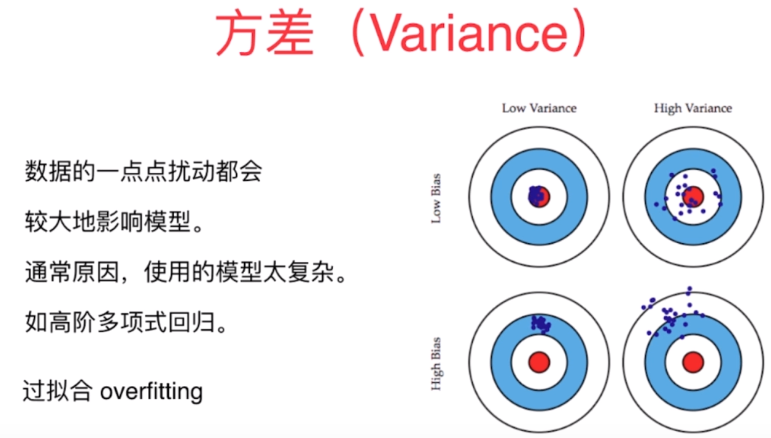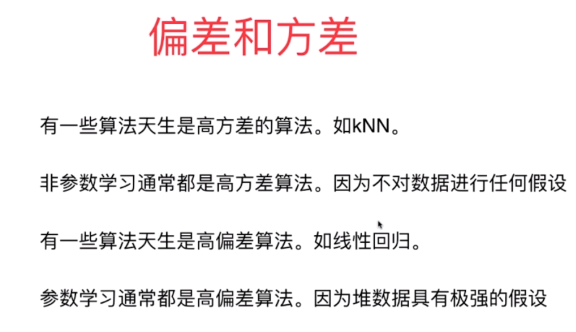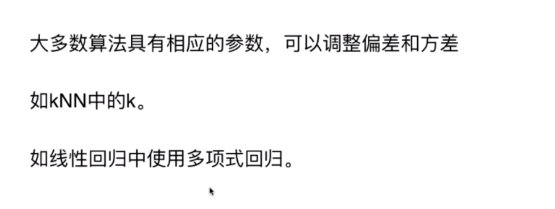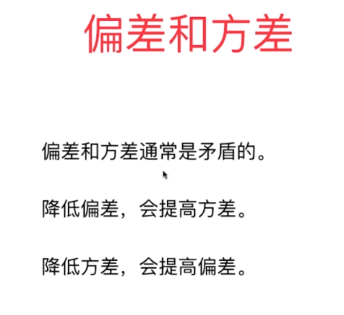假设数据来源可靠  特征明显    ==》机器学习算法 主要考虑方差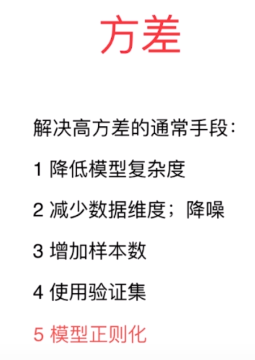模型正则化   ：系数越大曲线越陡峭==》让theta平方和小  theta0不在其中  theta0是截距

阿尔法是一个新的超参数=》决定theta平方和（尽可能小） 占整的损失函数的多少

阿尔法=0相当于没有加入正则化==》阿尔法无穷大 MS额E比重就比较小 此时的优化任务变为让每一个theta变小
我们要做的是要在预测准确度和让每个theta尽量小   两件事之间做一个平衡
方法：
岭回归
LASSO回归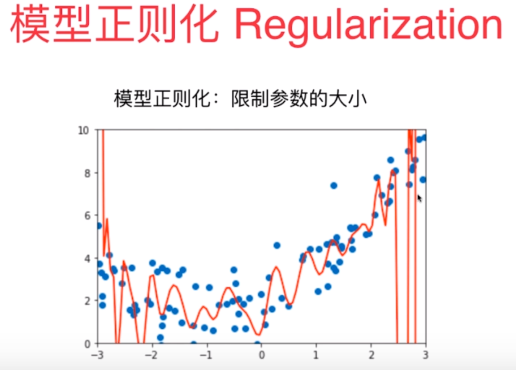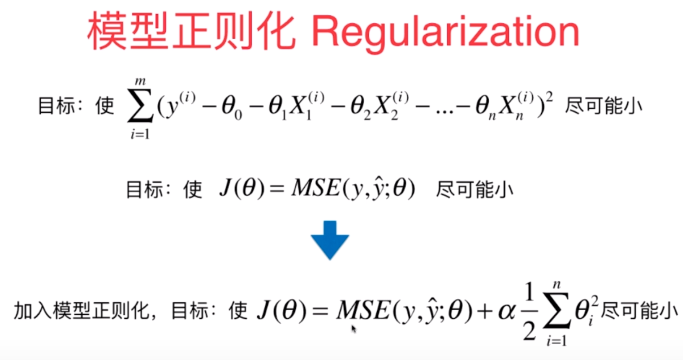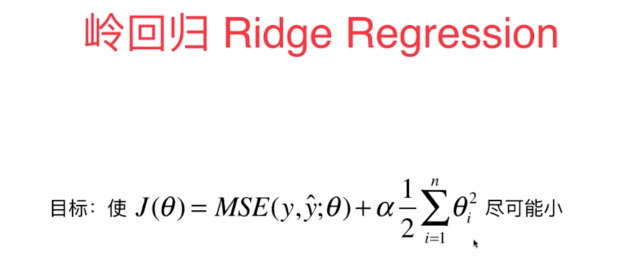岭回归过分 =》阿尔法取值过大就变成  是theta平方和最小 =》小到一定程度 为零 就成为了  线性函数 甚至平行于X轴
lasso回归：选择怎么表达theta最小上不一样  有降维作用
因为不想像回归逐渐的走 每个theta都是有数值的
lasso  |x|导数  = x/|x|  非0即+-1  沿着某一个方向一直走    直到一个theta为零换方向   继续直到直到最小值点
最后theta有可能很多维度上 达到零 （下面图有走的过程）

lasso回归缺点：有可能错误的将有用的特征前参数为0  使有用特征降掉    极易将某些元素化为0     一般从计算准确度上来讲还是岭回归比较好
优点：如果特征特别大 用多项回归degree要100  特征数非常多 此时使用lasso  也是非常好的  起到降维的作用  模型特征变小

选择顺序  岭回归  》 弹性网  》  lasso回归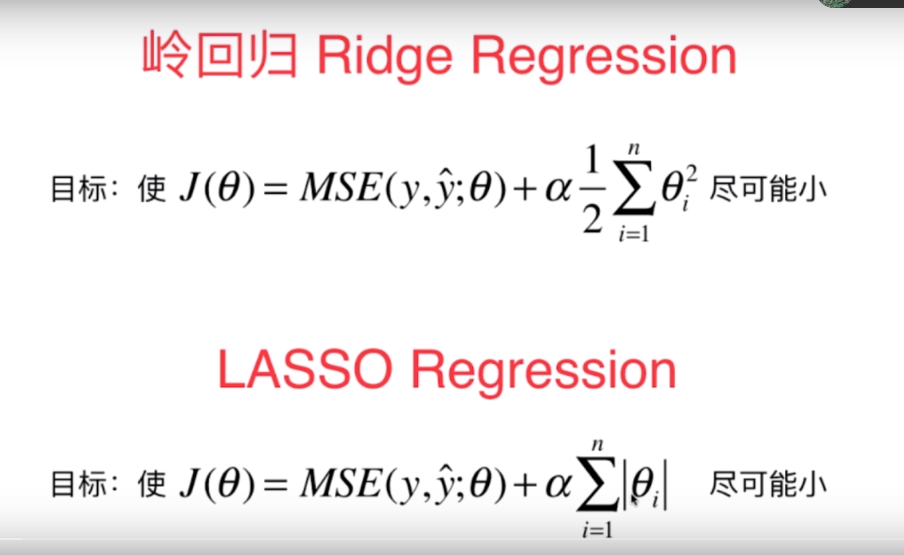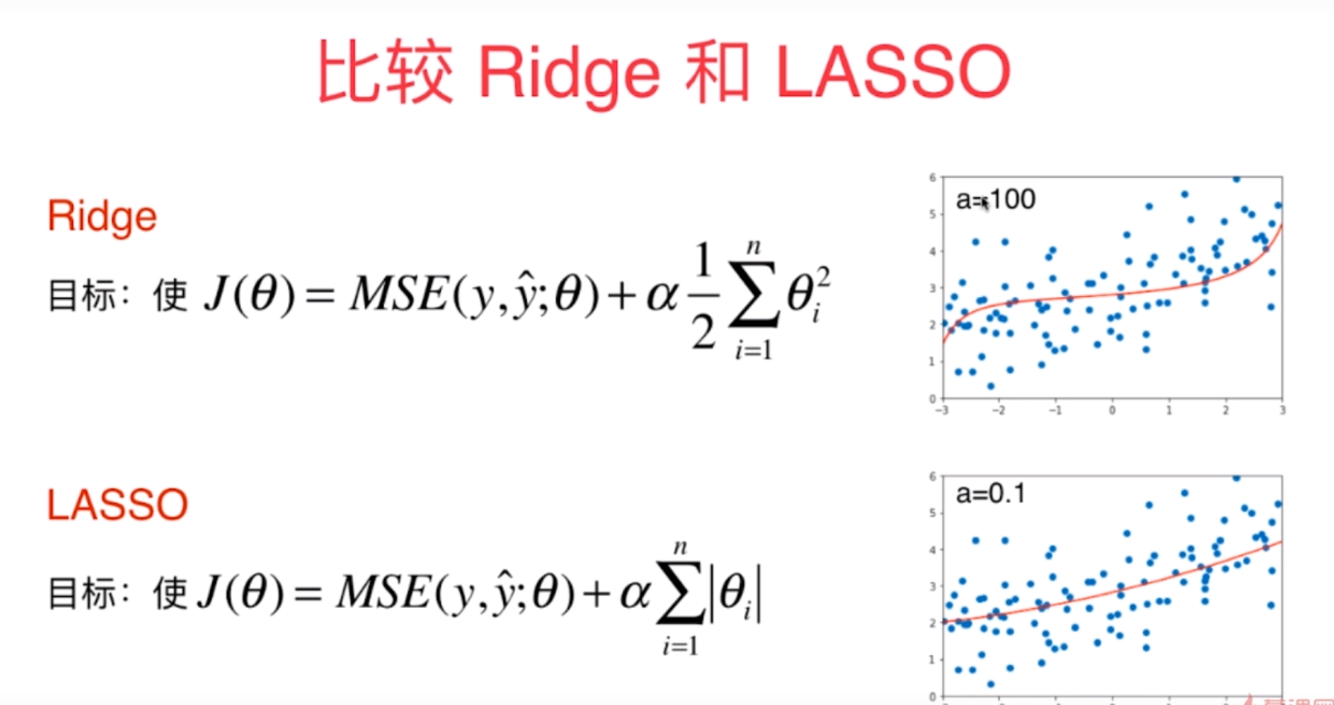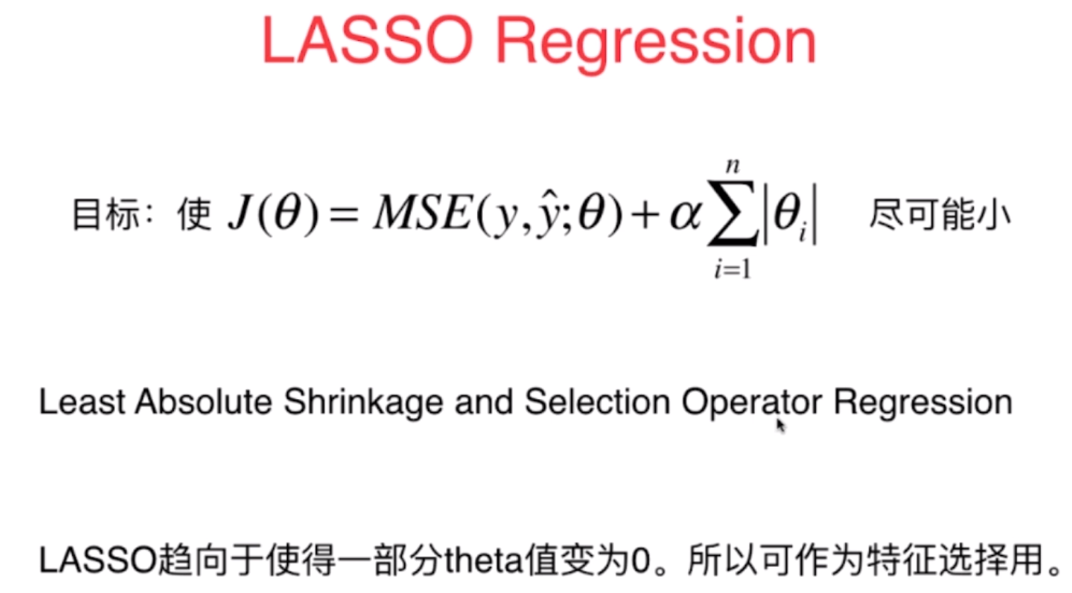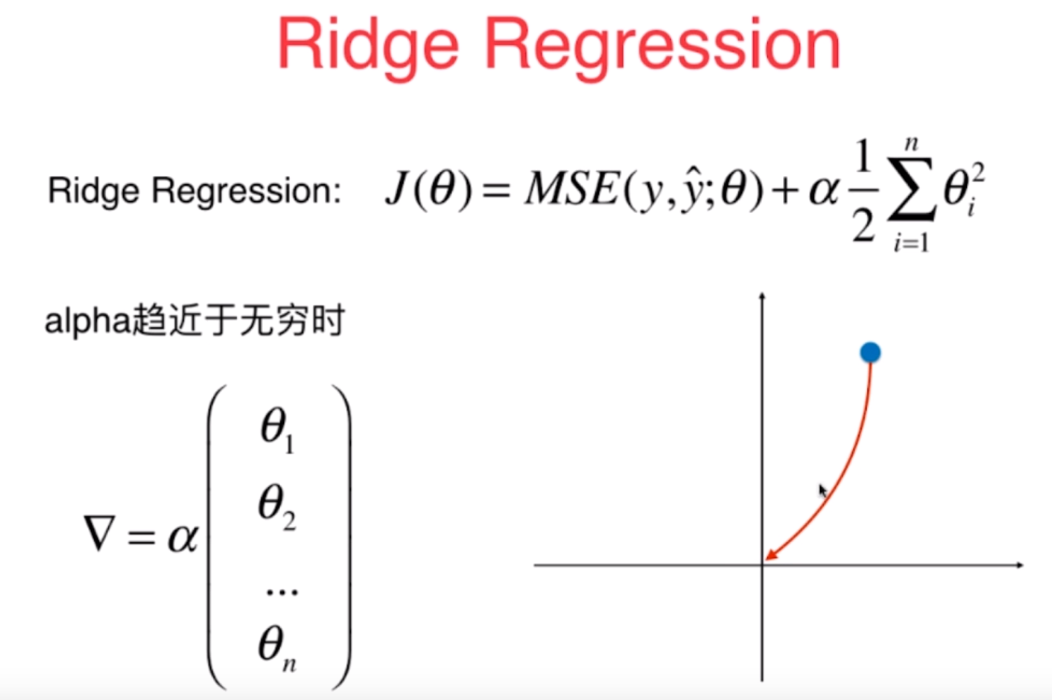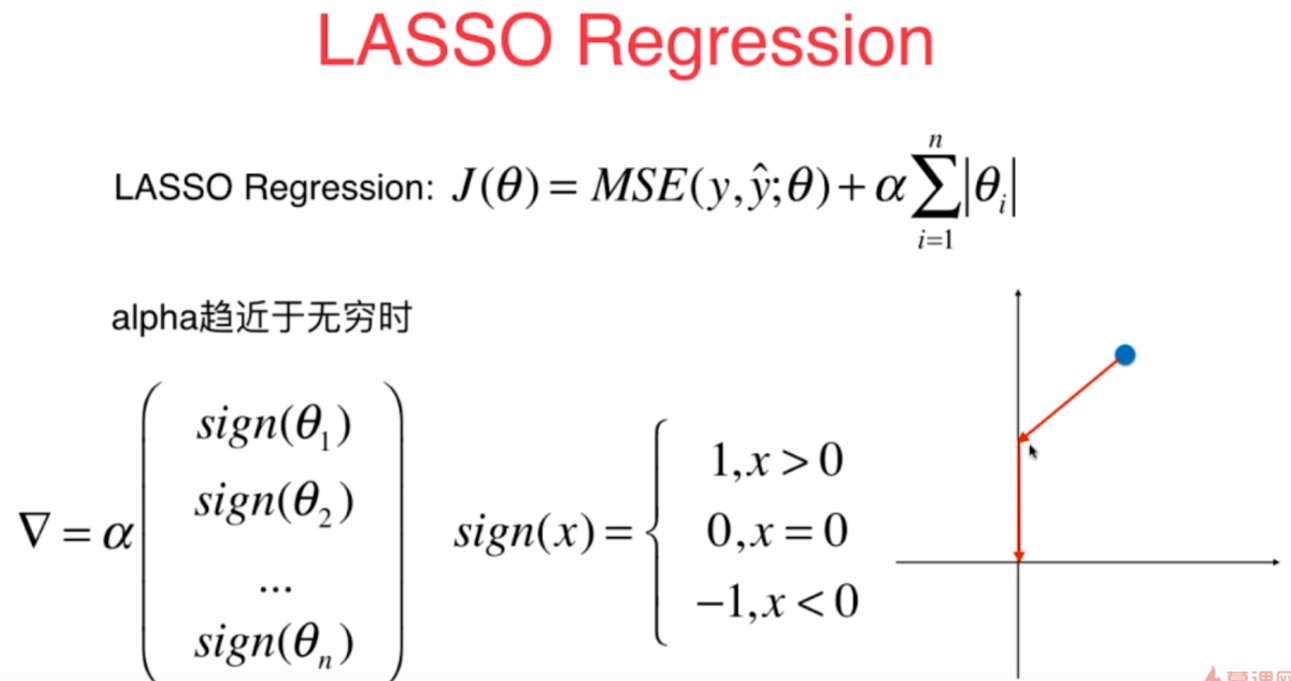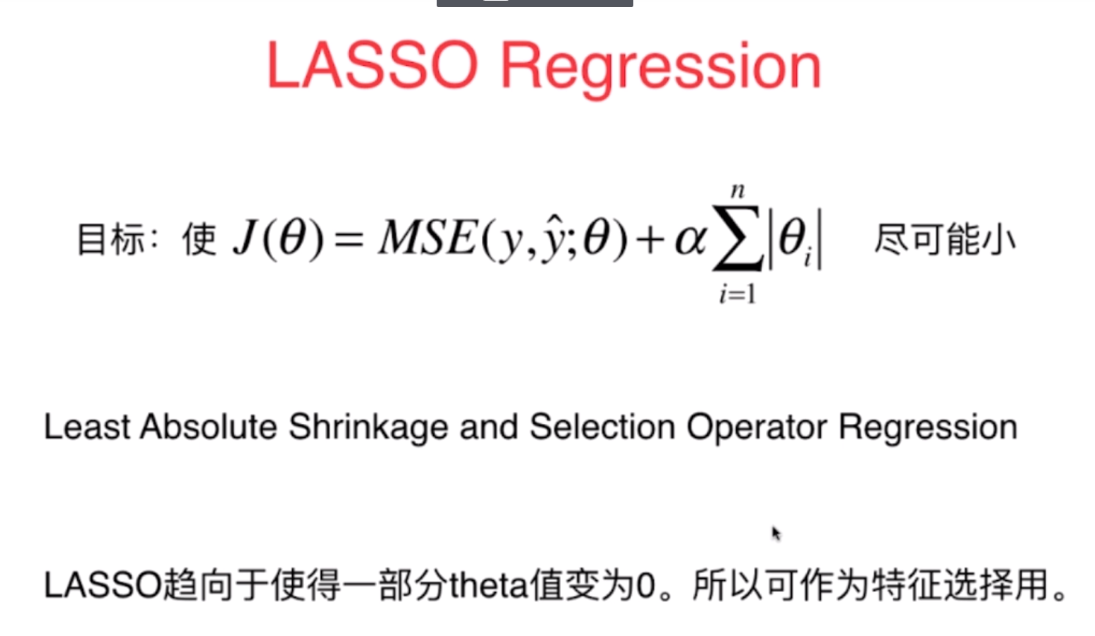无论是岭回归还是LASSO回归   两种模型正则化   都是在原始的损失函数后面添加一项     为了减小学习到的theta   使得模型的泛化能力变强    使模型系数不那么大 函数图像不那么怪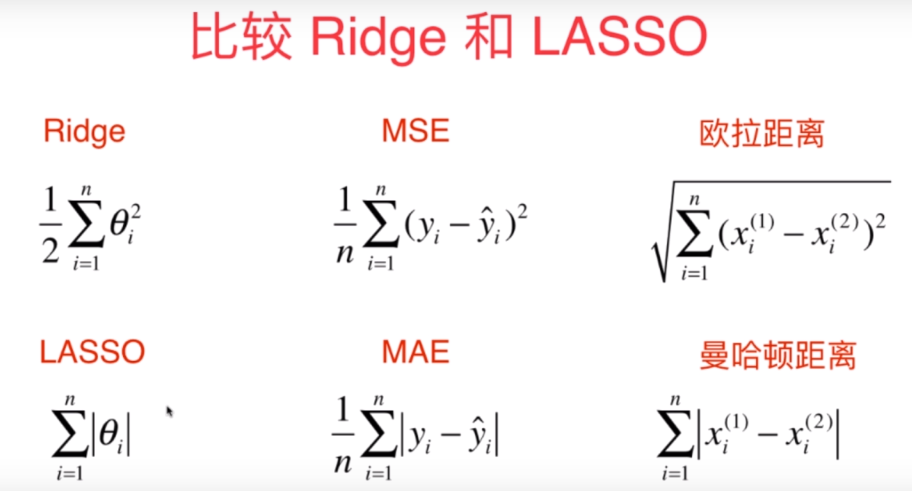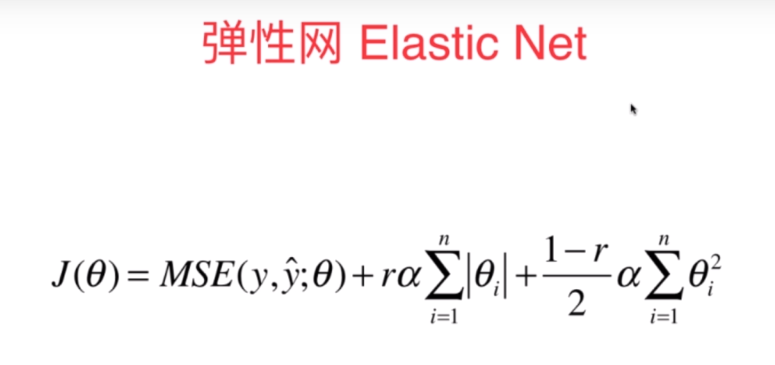展开全文• ## 多项式回归

万次阅读 多人点赞 2018-10-02 23:08:37
多项式回归模型是线性回归模型的一种，此时回归函数关于回归系数是线性的。由于任一函数都可以用多项式逼近，因此多项式回归有着广泛应用。 直线回归研究的是一个因变量与一个自变量之间的回归问题，但在实际情况中...
多项式回归
多项式回归，回归函数是回归变量多项式的回归。多项式回归模型是线性回归模型的一种，此时回归函数关于回归系数是线性的。由于任一函数都可以用多项式逼近，因此多项式回归有着广泛应用。
直线回归研究的是一个因变量与一个自变量之间的回归问题，但在实际情况中，影响因变量的自变量往往不止一个，例如：羊毛的产量受到绵羊体重、体长、胸围等影响，因此需要进行一个因变量与多个自变量间的回归分析，即多元回归分析。
研究一个因变量与一个或多个自变量间多项式的回归分析方法，称为多项式回归（Polynomial Regression）。如果自变量只有一个时，称为一元多项式回归；如果自变量有多个时，称为多元多项式回归。在一元回归分析中，如果依变量y与自变量x的关系为非线性的，但是又找不到适当的函数曲线来拟合，则可以采用一元多项式回归。
一元m次多项式回归方程为：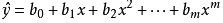二元二次多项式回归方程为：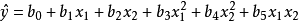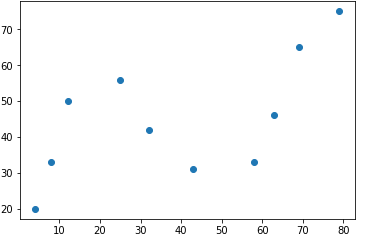上图的数据，我们可以使用一元2次多项式来拟合，首先，一个标准的一元高阶多项式函数如下：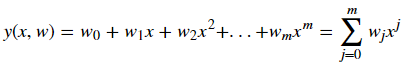m 表示多项式的阶数，表示 x 的 j 次幂，w 则代表该多项式的系数。
当我们使用上面的多项式去拟合散点时，需要确定两个要素，分别是：多项式系数 w 以及多项式阶数 m，这也是多项式的两个基本要素。当然也可以手动指定多项式的阶数m的大小，这样就只需要确定系数w的值了。得到以下公式：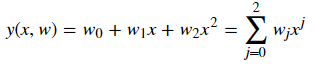如何求解该公式呢？这里使用Scipy的方法。
使用Scipy提供的最小二乘法函数得到最佳拟合参数：
该方法：最小化一组方程的平方和（即可以用来实现最小二乘法）
import numpy as np
from scipy.optimize import leastsq

# 拟合数据集
x = [4, 8, 12, 25, 32, 43, 58, 63, 69, 79]
y = [20, 33, 50, 56, 42, 31, 33, 46, 65, 75]

def fun(p, x):
"""
定义想要拟合的函数
"""
w0,w1 = p  #从参数p获得拟合的参数
# 如果是二次多项式则：w0,w1,w2 = p ；return w0 + w1*x + w2*x*x  以此类推
return w0 + w1*x

def err(p, x, y):
"""
定义误差函数
"""
return fun(p,x) -y

#定义起始的参数 即从 y = 1*x+1 开始，其实这个值可以随便设，只不过会影响到找到最优解的时间
p0 = [1,1]   #p0 = [1,1,1]    w系数的个数[w0,w1,w2...]

#将list类型转换为 numpy.ndarray 类型，最初我直接使用
#list 类型,结果 leastsq函数报错，后来在别的blog上看到了，原来要将类型转
#换为numpy的类型

x1 = np.array(x)
y1 = np.array(y)

xishu = leastsq(err, p0, args=(x1,y1))
print(xishu)
# xishu，即为获得的参数
一般只要指定前三个参数就可以：
func 是我们自己定义的一个计算误差的函数，  x0 是计算的初始参数值  args 是指定func的其他参数
通过实践后观察，上面实现1次多项式拟合（2次多项式，p0则需要3个值）但其效果都不是很好，所以下面修改代码尝试N(大于2)项拟合
"""
实现N次多项式拟合
"""
def fit_func(p, x):
"""根据公式，定义 n 次多项式函数
"""
f = np.poly1d(p)   # 这里的np.poly1d函数是用来构造多项式使用的，默认格式为:ax**2+bx+c等，如：ax**3 + bx**2 + cx + d 以此类推
return f(x)

def err_func(p, x, y):
"""残差函数（观测值与拟合值之间的差距）
"""
ret = fit_func(p, x) - y
return ret

def n_poly(n):
"""n 次多项式拟合
"""
p_init = np.random.randn(n) # 生成 n 个随机数
parameters = leastsq(err_func, p_init, args=(np.array(x), np.array(y)))
return parameters	# 返回多项式系数w0、w1、w2...

k = n_poly(3)	# 与上面的二次多项式结果一致，只是公式顺序不同

"""绘制出 3，4，5，6，7, 8 次多项式的拟合图像
"""
# 绘制拟合图像时需要的临时点
x_temp = np.linspace(0, 80, 10000)
# 绘制子图
fig, axes = plt.subplots(2, 3, figsize=(15,10))

axes[0,0].plot(x_temp, fit_func(n_poly(4), x_temp), 'r')
axes[0,0].scatter(x, y)
axes[0,0].set_title("m = 3")

axes[0,1].plot(x_temp, fit_func(n_poly(5), x_temp), 'r')
axes[0,1].scatter(x, y)
axes[0,1].set_title("m = 4")

axes[0,2].plot(x_temp, fit_func(n_poly(6), x_temp), 'r')
axes[0,2].scatter(x, y)
axes[0,2].set_title("m = 5")

axes[1,0].plot(x_temp, fit_func(n_poly(7), x_temp), 'r')
axes[1,0].scatter(x, y)
axes[1,0].set_title("m = 6")

axes[1,1].plot(x_temp, fit_func(n_poly(8), x_temp), 'r')
axes[1,1].scatter(x, y)
axes[1,1].set_title("m = 7")

axes[1,2].plot(x_temp, fit_func(n_poly(9), x_temp), 'r')
axes[1,2].scatter(x, y)
axes[1,2].set_title("m = 8")
plt.show()
很简单，代码分为两部分，上面为主要的计算系数w的值，下面为直观查看每个次项拟合后的效果图，如下：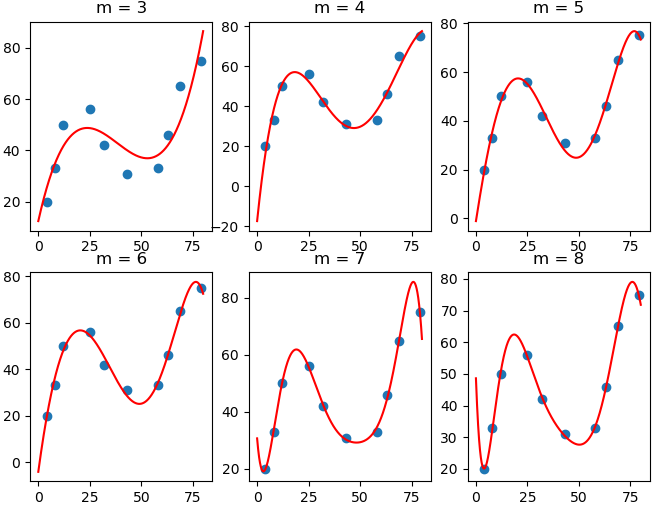可以清楚的看到当3次项（也就是m=3）时，效果还是一般，但从4次项（m=4）后，对于数据的拟合性就明显优于3次项了，当 m=8 时，曲线呈现出明显的震荡，这也就是线性回归实验中所讲到的过拟和（Overfitting）现象，后面再介绍如何解决这个问题。
使用scikit-learn进行多项式拟合：
对于一个二次多项式而言，我们知道它的标准形式为：，但是，多项式回归其实相当于是线性回归的特殊形式（开头也提到）。例如，我们这里令，，那么原方程就转换为：，这也就变成了多元线性回归。完成了一元高次多项式到多元一次项式之间的转换。（如下，看作将多元一次项合并为一个矩阵中线性求解）
举例说明，对于自变量向量  和因变量 ，如果 ：
[[ 3]                  [-2]                        [ 4]]
则可以通过线性回归模型进行拟合。同样，如果对于一元二次多项式，能得到，构成的特征矩阵，即：
[[ 3.  9.]                 [-2.  4.]                                          [ 4. 16.]]
那么也就可以使用线性回归进行拟合了。
所以这里有了一个方便的工具，scikit-learn 中，我们可以通过 PolynomialFeatures() 类自动产生多项式特征矩阵

sklearn.preprocessing.PolynomialFeatures(degree=2, interaction_only=False, include_bias=True)

degree: 多项式次数，默认为 2 次多项式  interaction_only: 默认为 False，如果为 True 则产生相互影响的特征集。  include_bias: 默认为 True，包含多项式中的截距项。
通过下面代码解决上面一样的问题：
"""
使用 PolynomialFeatures 自动生成特征矩阵
"""
from sklearn.preprocessing import PolynomialFeatures

x = np.array(x).reshape(len(x), 1) # 转换为列向量
y = np.array(y).reshape(len(y), 1)

poly_features = PolynomialFeatures(degree=2, include_bias=False)    # 特征矩阵模型构建用来多项式的特征矩阵
poly_x = poly_features.fit_transform(x)    # 通过模型转换x数据

"""
用线性回归拟合上面转换后的数据
"""
from sklearn.linear_model import LinearRegression

model = LinearRegression()
model.fit(poly_x,y)		# 这里使用转换后的特征矩阵相当于将一元2次转换为二元一次
# print(model.intercept_,model.coef_)

"""绘制拟合图像
"""
x_temp = np.linspace(0, 80, 10000)
x_temp = np.array(x_temp).reshape(len(x_temp),1)
poly_x_temp = poly_features.fit_transform(x_temp)

plt.plot(x_temp, model.predict(poly_x_temp), 'r')
plt.scatter(x, y)
plt.show()         # 得到的结果与上面用leastsq的二次项结果一致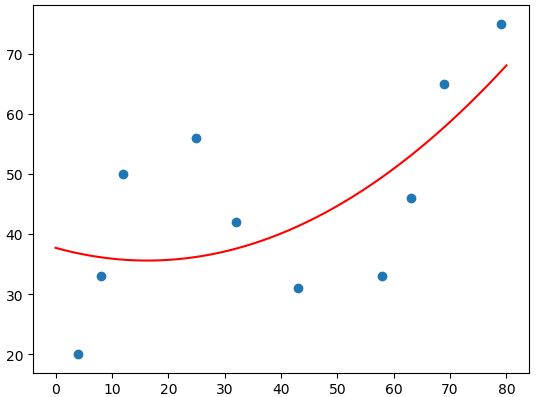这里二次项的图（degree=2），若设置更高的次项3，4，5（degree=3，4，5），则可以得到上面leastsq方法相同的效果，只需要通过PolynomialFeatures方法生成对应次项的特征矩阵就行。
评价指标
可以使用前一篇文章提到的 平均绝对误差（MAE）、均方误差（MSE）等方法来衡量，具体可以使用sklearn中的以下两个方法：

from sklearn.metrics import mean_absolute_error from sklearn.metrics import mean_squared_error

2次多项式不一定比线性回归的效果好，需要根据具体的测试结果做决定，可以试试更高次的多项式回归预测 ，下面我们便来验证以一下。
make_pipeline通道：
通过实例化 make_pipeline 管道类，实现调用一次 fit 和 predict 方法即可应用于所有预测器。make_pipeline 是使用 sklearn 过程中的技巧创新，其可以将一个处理流程封装起来使用。 更详细的使用可以参考这里
上面的多项式回归中，我们需要先使用 PolynomialFeatures 完成特征矩阵转换，再放入 LinearRegression 中。那么，PolynomialFeatures + LinearRegression 这一个处理流程，就可以通过 make_pipeline 封装起来使用。
以下数据集并非上面的x，y，如下数据（将Year作为特征列train_x，Values为目标值train_y，且数据是按0.7比例分割为训练、测试集）：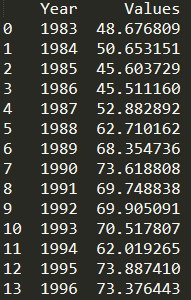"""更高次多项式回归预测
"""
from sklearn.pipeline import make_pipeline

train_x = train_x.reshape(len(train_x),1)
test_x = test_x.reshape(len(test_x),1)
train_y = train_y.reshape(len(train_y),1)

for m in [3, 4, 5]:
model = make_pipeline(PolynomialFeatures(m, include_bias=False), LinearRegression())
model.fit(train_x, train_y)
pre_y = model.predict(test_x)
print("{} 次多项式回归平均绝对误差: ".format(m), mean_absolute_error(test_y, pre_y.flatten()))
print("{} 次多项式均方根误差: ".format(m), mean_squared_error(test_y, pre_y.flatten()))
print("---")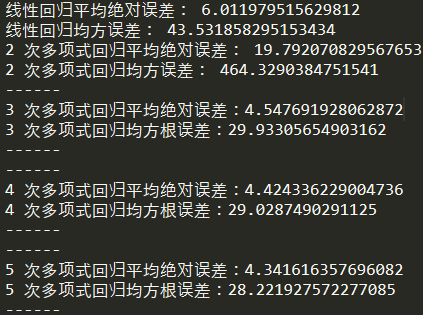可以看到高次项的误差都比线性回归要低，而2次项则要高，所以对于模型均需要进行验证后使用。
好了，上面介绍了 多项式回归的原理与Scipy实现、sklearn构建特征矩阵转为线性运算、Pipeline方便的通道运算、以及误差评判，但还有一个很重要的问题，那就是如何选择最佳的n次项？
几次项才是最佳的选择？
其实这个问题很简单，我们可以设置一个误差指标（MSE、MAE等），然后绘制增加次项后，预测结果的误差值图。观察图形来选择一个合适的次项点（类似肘部法则也可以作为参考）：
"""
计算 m 次多项式回归预测结果的 MSE 评价指标并绘图
"""
mse = [] # 用于存储各最高次多项式 MSE 值
m = 1 # 初始 m 值
m_max = 10 # 设定最高次数
while m <= m_max:
model = make_pipeline(PolynomialFeatures(m, include_bias=False), LinearRegression())
model.fit(train_x, train_y) # 训练模型
pre_y = model.predict(test_x) # 测试模型
mse.append(mean_squared_error(test_y, pre_y.flatten())) # 计算 MSE
m = m + 1

# print("MSE 计算结果: ", mse)
# 绘图
plt.plot([i for i in range(1, m_max + 1)], mse, 'b')
plt.scatter([i for i in range(1, m_max + 1)], mse)

# 绘制图名称等
plt.title("MSE of m degree of polynomial regression")
plt.xlabel("m")
plt.ylabel("MSE")
plt.show()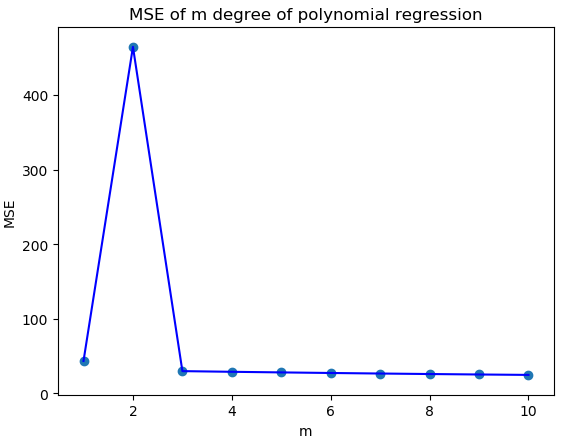如上图，可以明显看到当次数为3时，误差指标已经趋近于平稳了，所以这里选择3最好，当次数项越多可能会出现过拟合的问题，模型的泛化能力会降低。

参考文章：
https://blog.csdn.net/lanchunhui/article/details/50521648
展开全文Scipy
• 首先在文件lorenza.dat中读取出前20个数据，然后将这20个数据代入到公式中求出多项式系数，从而建立出多项式回归模型，最后通过建立出来的多项式回归模型画出P次多项式的数据拟合图。 # -*- coding: utf-8 -*- """ ...python
• 凌云时刻 · 技术导读：在前面的笔记中，我们使用的样本数据都是具有一定线性关系的，我们使用简单线性回归或者多元线性回归拟合这些数据。但是这种具有强假设的数据集在实际应用中是比较少的，大...
• python多项式回归Let’s start with an example. We want to predict the Price of a home based on the Area and Age. The function below was used to generate Home Prices and we can pretend this is “real-...
• 多项式回归是一种线性回归形式，其中自变量x和因变量y之间的关系被...如果我们尝试将线性模型拟合到曲线数据，则预测变量（X轴）上的残差（Y轴）的散点图将在中间具有许多正残差的斑块。因此，在这种情况下，这是不...Python
• 之前我们曾经学习了简单线性回归模型的推导、sklearn实战，并尝试从零搭建了一个简单线性回归的模型工具。 但是我们遇到的数据并不总是线性的，这时如果我们还拿线性模型去拟合，我们模型的效果就会大打折扣。不过...机器学习 线性回归 人工智能
• 机器学习算法之多项式回归 目录: 1.多项式回归简介 2.编程实验多项式回归 3.过拟合和欠拟合 4.学习曲线 5.验证数据集与交叉验证 6.偏差方差均衡 7.模型正则化 一、多项式回归简介 考虑下面的数据，虽然我们可以...机器学习算法
• 最后可能是这样的情况，总成本不再是数量的线性函数： 通过多项式回归，我们可以将n阶模型拟合到数据上，并尝试对非线性关系进行建模。 如何拟合多项式回归 这是我们模拟观测数据的图。模拟的数据点是蓝色的点，而...
• return torch.mm(x, w) + b #画出更新之前的模型 y_pred = multi_linear(x_train) plt.plot(x_train.data.numpy()[:, 0], y_pred.data.numpy(), label='fitting curve',color='r')#X[:,0]就是取所有行的第0个数据 ...
• 有时候，多项式回归会得出拟合效果更好的模型，但是也需要注意过拟合的线性。 下面，还是以房屋面积预测房屋价格的数据为例： 读取数据，绘制散点图： 多项式回归 import matplotlib.font_manager as fm import...
• 什么是多项式回归 多项式回归思路与多元线性回归相似，只是为原来的数据样本添加新的特征，而新的特征是原有特征的多项式组合。 scikit-learn中的多项式回归与pipeline ...岭回归 LASSO回归
• 4）三阶多项式函数模型拟合 n_train_two = 2 from sklearn . linear_model import LinearRegression from sklearn . preprocessing import PolynomialFeatures ploy_reg = PolynomialFeatures ( ...深度学习 sklearn
• 对于一般的线性回归模型，由于该函数拟合出来的是一条直线，所以精度欠佳，我们可以考虑多项式回归，构造非线性特征，用的是高次多项式而不是简单的一 次线性多项式。所谓多项式回归，其本质也是线性回归。也就是说...
• 多项式回归是一种线性回归形式，其中自变量x和因变量y之间的关系被建模为n次多项式。...在多项式回归模型中，不满足该假设。 多项式回归的使用： 这些基本上用于定义或描述非线性现象，例如： 组织生长速度。 疾病
• print("2次多项式回归模型的性能评估：",regressor_poly2.score(x_test_poly2, y_test)) x_test_poly4 = poly4.transform(x_test) print("4次多项式回归模型的性能评估：",regressor_poly4.score(x_test_poly4, y_...
• 对于所有的线性回归模型（包括多项式回归），我们都可以用最小二乘法或者梯度下降法去求解，这两种算法各有利弊，其中： 对于最小二乘法来说，它是利用线性代数里求解矩阵方程的方法得到参数向量，不需要多次迭代，...python 算法 随机梯度下降 numpy 机器学习
• 多项式回归模型正则化（拉索，岭，弹性网络） 目录多项式回归模型正则化（拉索，岭，弹性网络）一、pandas是什么？二、使用步骤1.引入库2.读入数据总结 提示：这里可以添加本文要记录的大概内容： 例如：随着人工...机器学习 python
• 1、多元线性回归 n 个预测器变量，那么模型可以用以下方程...你将用一个模型拟合这 13 个特征，以预测房价 from sklearn.linear_model import LinearRegression from sklearn.datasets import load_boston # Load t...机器学习
• 一. 定义 我们实际希望的，是在新样本上能表现得很好的学习器。为了达到这个目的，应该从训练样本中尽可能学出适用于所有潜在样本的“普遍规律”，这样才能在遇到新样本时做出正确的判别....与"过拟合"相对的是“...
• 拟合和欠拟合拟合underfitting 算法所训练的模型不能完整...红线表明了，随着模型复杂度的提高，会出现由欠拟合到过拟合的变化 学习曲线 随着训练样本的逐渐增多，算法训练出的模型的表现能力 构造数据 In [...机器学习
• 多项式曲线拟合（Polynomial Curve Fitting）标签：监督学习@ author : duanxxnj@163.com @ time : 2016-06-19原文链接多项式特征生成在机器学习算法中，基于针对数据的非线性函数的线性模型是...关于多项式回归的...
• PyTorch基础入门三：PyTorch搭建多项式回归模型  1）理论简介 对于一般的线性回归模型，由于该函数拟合出来的是一条直线，所以精度欠佳，我们可以考虑多项式回归来拟合更多的模型。所谓多项式回归，其本质也是...人工智能 深度学习 PyTorch
• 拟合和欠拟合(overfitting and underfitting) ...下面通过一个多项式回归的例子来感性的认识这两个概念： Step1 随机生成一组具有非线性关系的数据集合 import numpy as np import matplotlib.pypl机器学习 python...